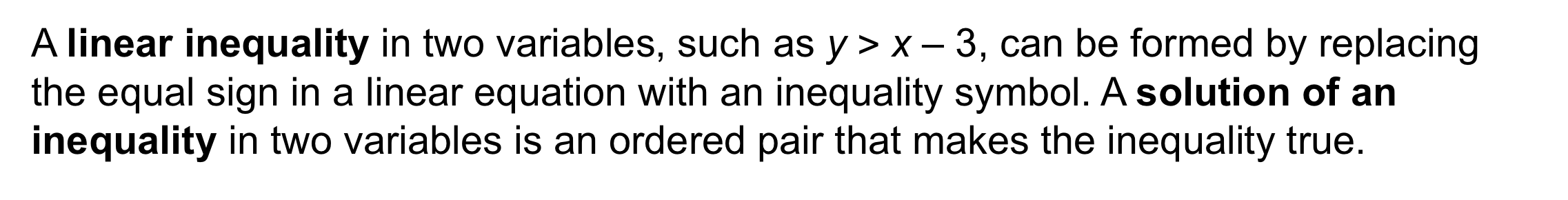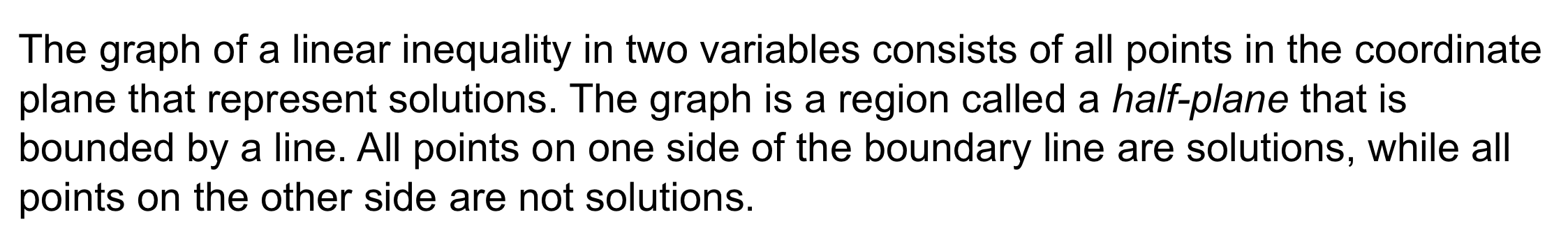Algebra 1 6-5 Complete Lesson: Linear Inequalities
starstarstarstarstarstarstarstarstarstar
5 (1 rating)
by Matthew Richardson
| 22 Questions
Note from the author:
A complete formative lesson with embedded slideshow, mini lecture screencasts, checks for understanding, practice items, mixed review, and reflection. I create these assignments to supplement each lesson of Pearson's Common Core Edition Algebra 1, Algebra 2, and Geometry courses. See also mathquest.net and twitter.com/mathquestEDU.The outlined content above was added from outside of Formative.1
1
10 pts
Solve It! You are buying paperback and hardcover books at a book sale. You can spend at most \$20. What are the possible combinations of paperback and hardcover books that you can buy? Explain.2
2
5 pts
Problem 1 Got It?
A
B3
3
5 pts
Problem 1 Got It?
A
B4
10 pts
Problem 2 Got It? What is the graph of the inequality below?
5
10 pts
Problem 3 Got It? What is the graph of the inequality?

6
10 pts
Problem 3 Got It? What is the graph of the inequality?7
10 pts
Problem 4 Got It? For a party, you can spend no more than \$12 on nuts. Peanuts cost \$2/lb. Cashews cost \$4/lb. What are three possible combinations of peanuts and cashews you can buy. Graph the inequality.8
8
10 pts
Problem 5 Got It?
A
B
C
D9
10 pts
Is (-1, 4) a solution of the inequality? Explain.

No. Substituting the ordered pair (-1, 4) into the inequality makes it false.
Yes. Substituting the ordered pair (-1, 4) into the inequality makes it true.
The outlined content above was added from outside of Formative.
10
10 pts
Graph the linear inequality on the canvas below by hand, without the aid of a graphing utility.
After that, graph the inequality on the embedded Desmos graphing calculator above.
As always, include important graph detail: label the axes, indicate axis scale by labeling at least one value on each axis, add arrows to the ends of lines, ensure that your line is appropriately dashed or solid, and shade one side of the boundary line, as appropriate.
11
10 pts
Graph the linear inequality. Zoom and pan your graph to establish an appropriate viewing window.12
12
10 pts
What is an inequality that represents the graph shown?
A
B
C
D13
10 pts
Vocabulary: How is a linear inequality in two variables like a linear equation in two variables? How are they different?
14
10 pts
Writing: To graph the inequality below, do you shade above or below the boundary line? Explain.

Below; The inequality sign < indicates all values less than. y-values decrease as you move down a coordinate plane.
Above; The inequality sign < indicates all values less than. y-values decrease as you move down a coordinate plane.
15
10 pts
Reasoning: Write an inequality that describes the region of the coordinate plane not included in the graph of the inequality below.16
10 pts
Review Lesson 6-4: An electrician spends \$12,000 on initial costs to start a new business. He estimates his expenses at \$25 per day. He expects to earn \$150 per day. If his estimates are correct, after how many working days will he break even? Enter only a number.
17
10 pts
Review Lesson 3-6: What compound inequality represents the phrase "all real numbers that are greater than 2 and less than or equal to 7"?
2 < x ≤ 7
2 ≤ x < 7
2 > x ≤ 7
18
10 pts
Review Lesson 6-1: Solve the system by graphing. Tell whether the system has one solution, infinitely many solutions, or no solution.19
10 pts
Vocabulary Review A solution is a value or values which, when substituted for a variable, make an equation true. Determine the solution of each inequality below.
• Solution: x > 20
• Solution: x < 20
• Solution: m > 6
• Solution: m < 6
• x + 17 < 37
• 2m + 3 > 1520
10 pts
Use Your Vocabulary: Identify the linear inequality in two variables.

21
100 pts
Notes: Take a clear picture or screenshot of your Cornell notes for this lesson. Upload it to the canvas. Zoom and pan as needed.